# RD Sharma Solutions for Class 9 Maths Chapter 18 Surface Area and Volume of Cuboid and Cube Exercise 18.2

Class 9 chapter 18 – Surface Area and Volume of Cuboid and Cube Exercise 18.2 solutions are provided only here. The RD Sharma Maths Solutions for Class 9 are the best and the most effective tool to prepare Maths for class 9. Exercise 18.2 solutions are provided here to help students understand the topic – Surface Area and Volume of Cuboid and Cube in an easy manner. In this exercise, students will learn about the volume of a cuboid and cube.

## Download PDF of RD Sharma Solutions for Class 9 Maths Chapter 18 Surface Area and Volume of Cuboid and Cube Exercise 18.2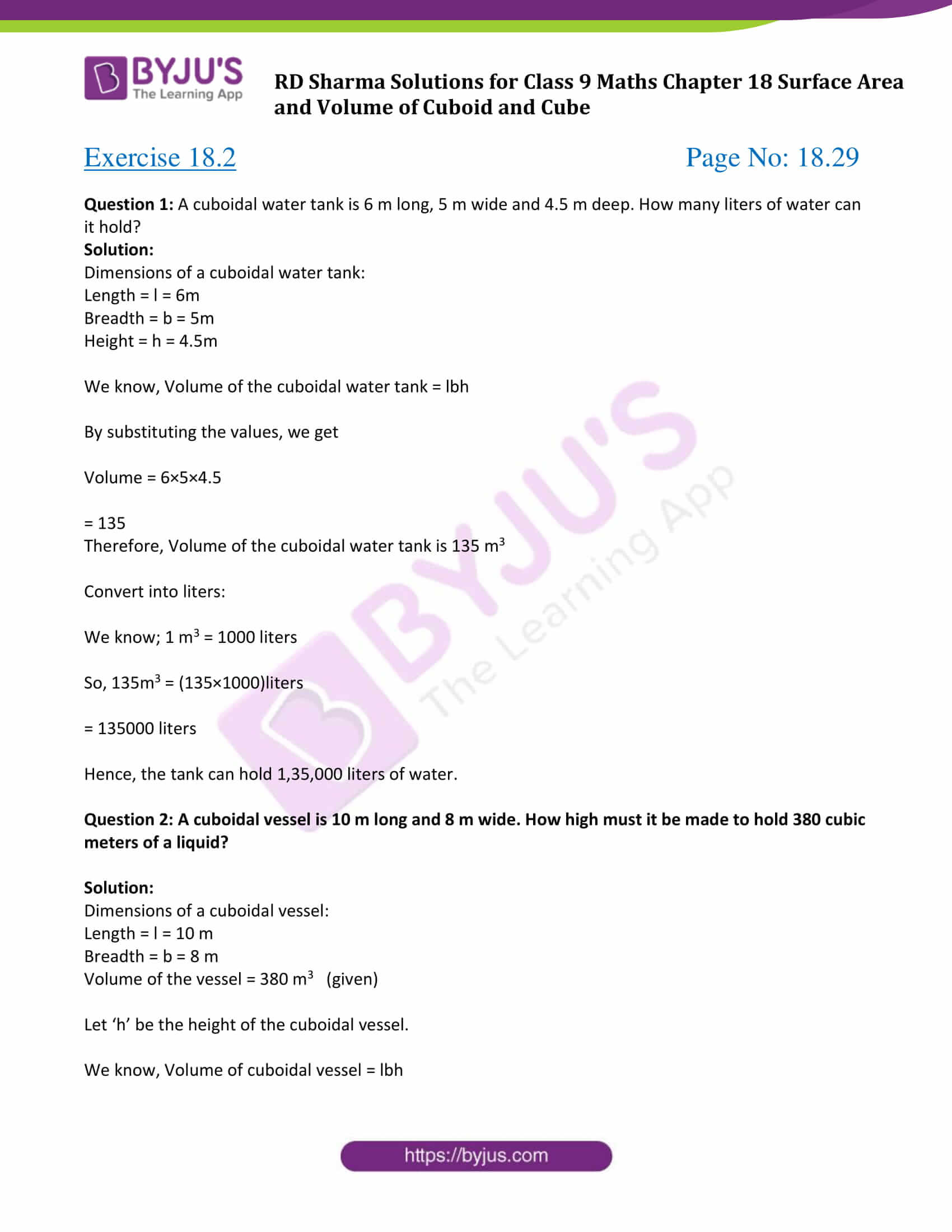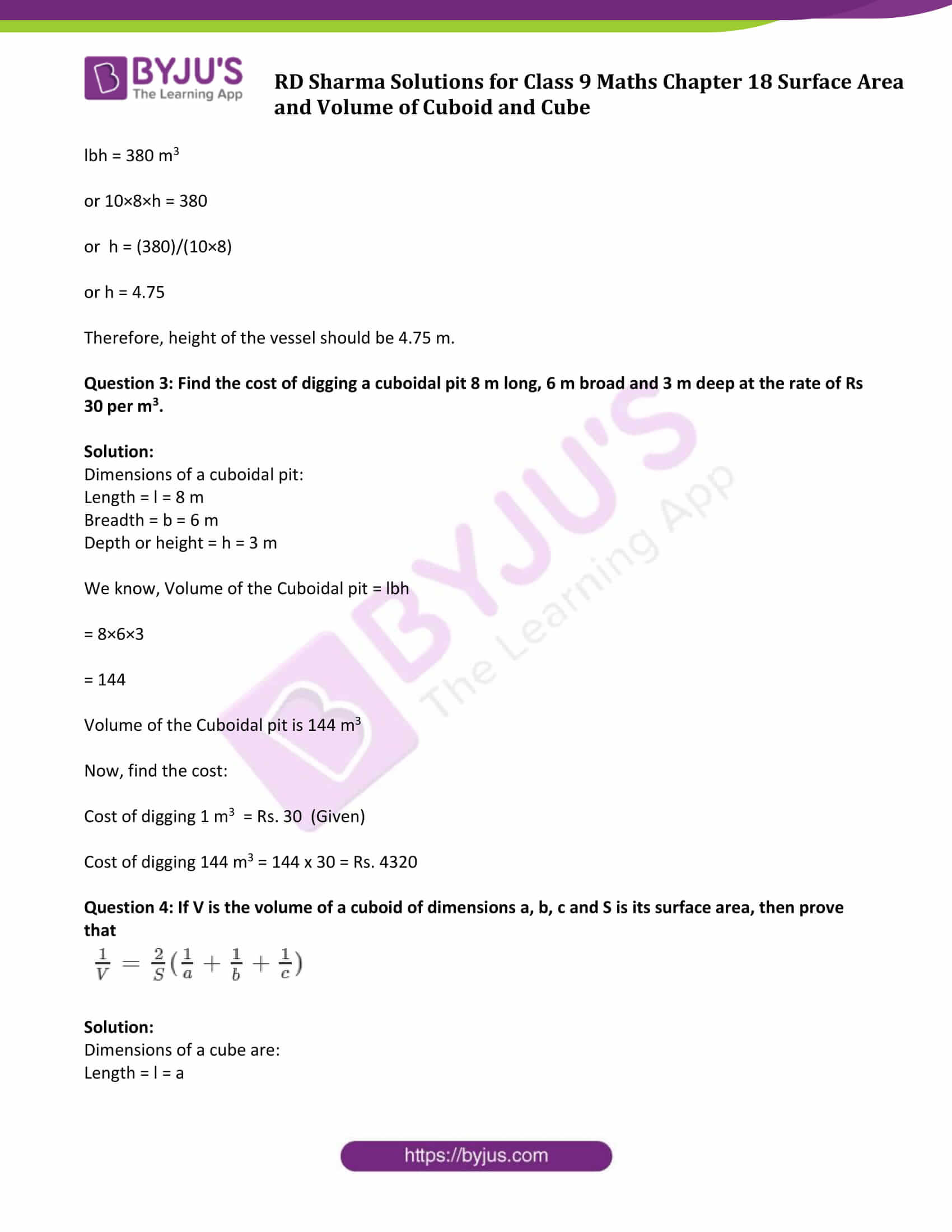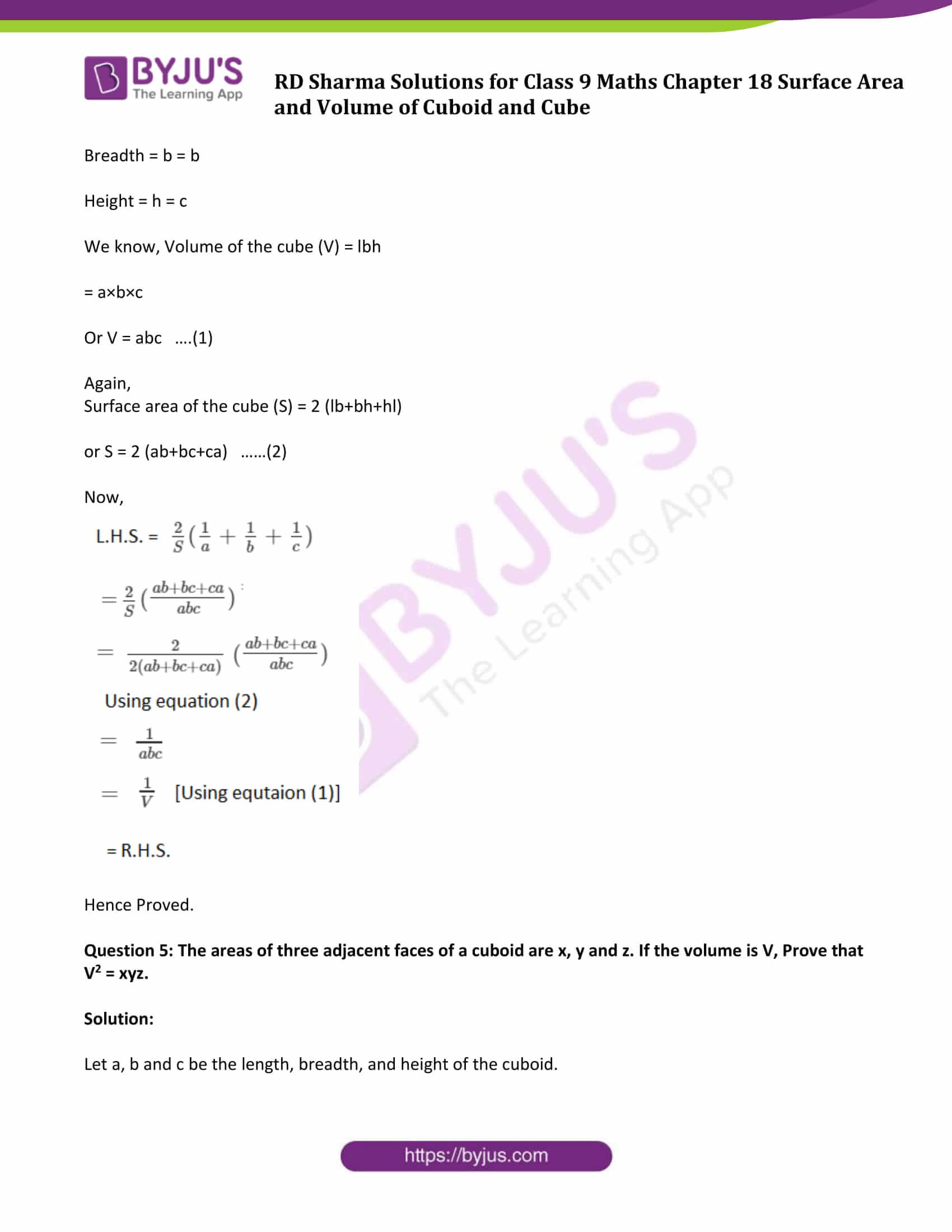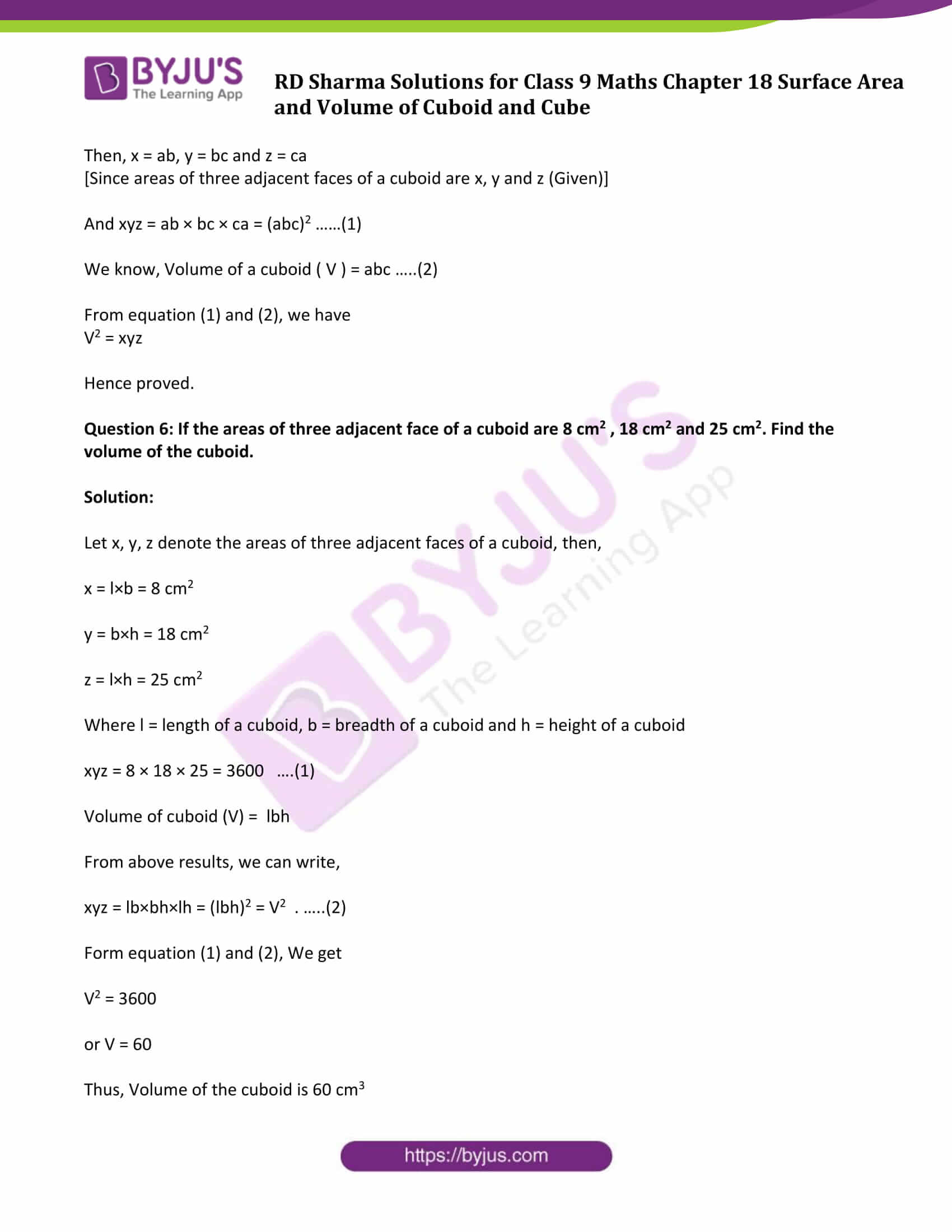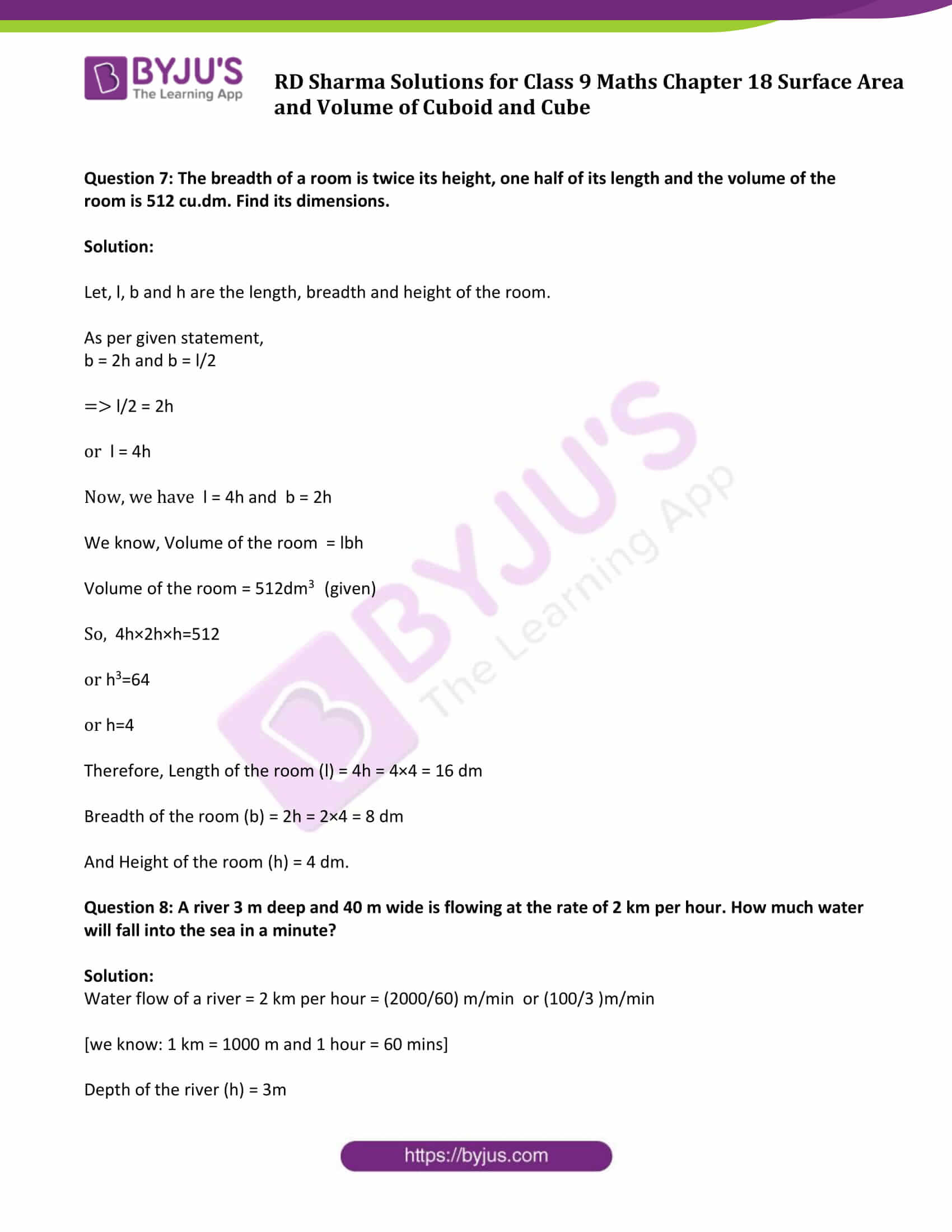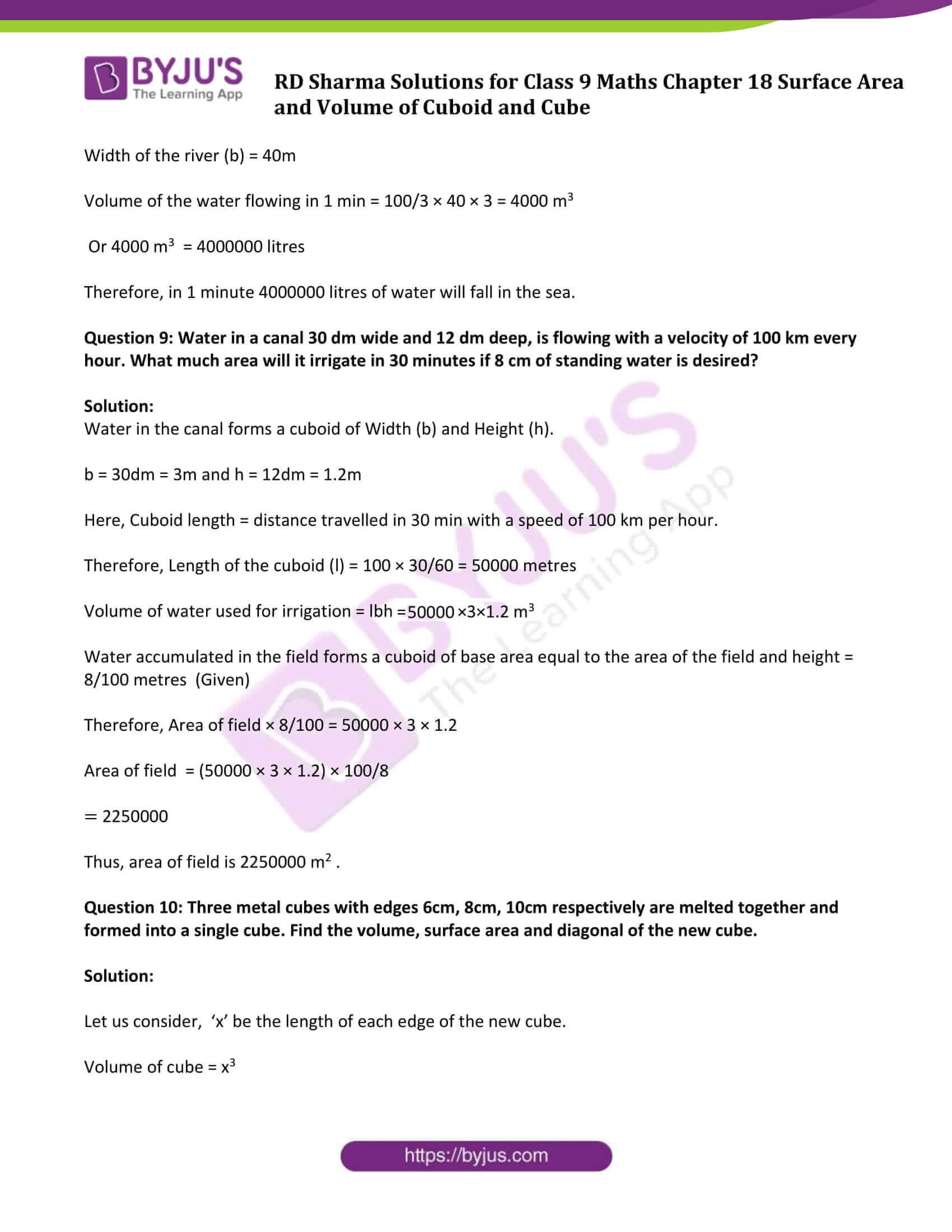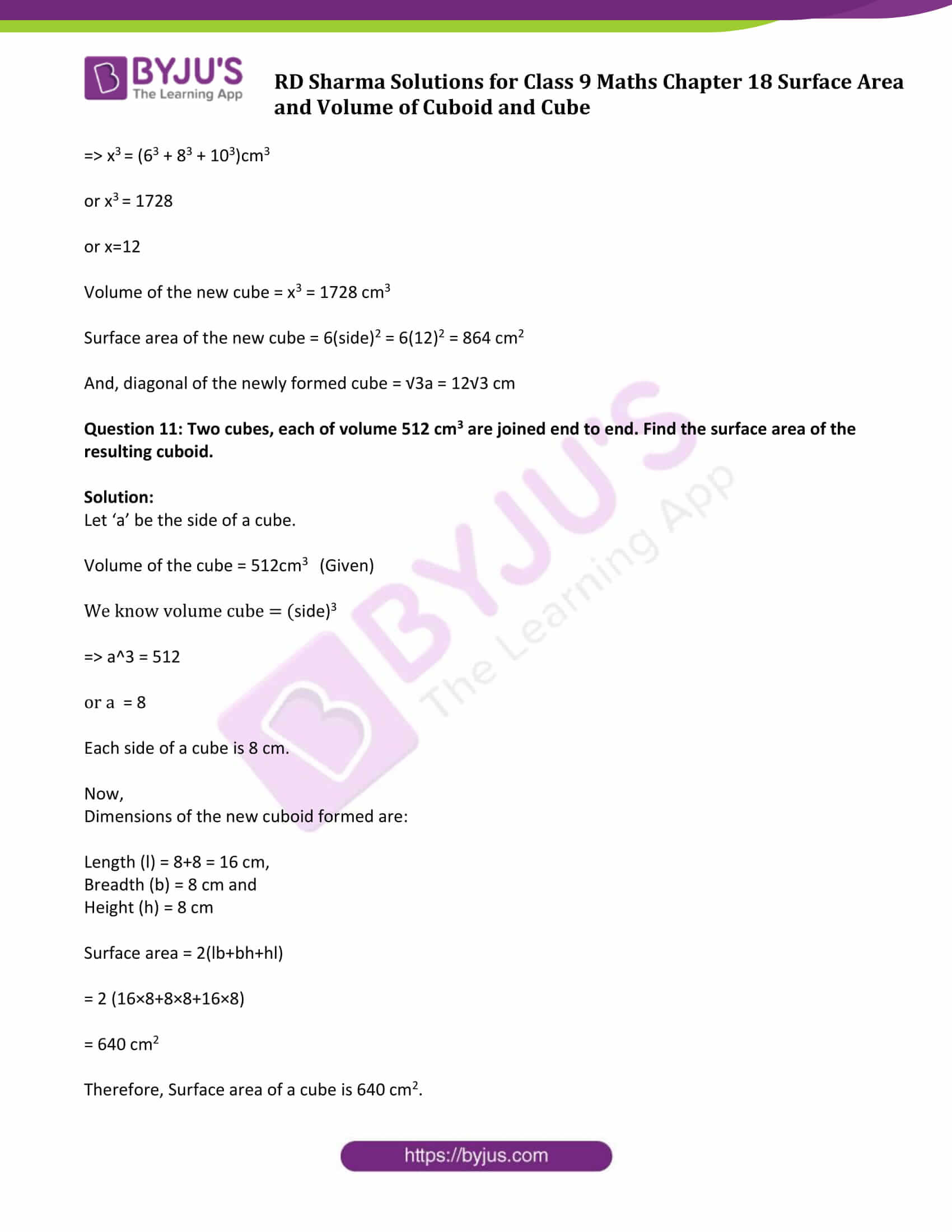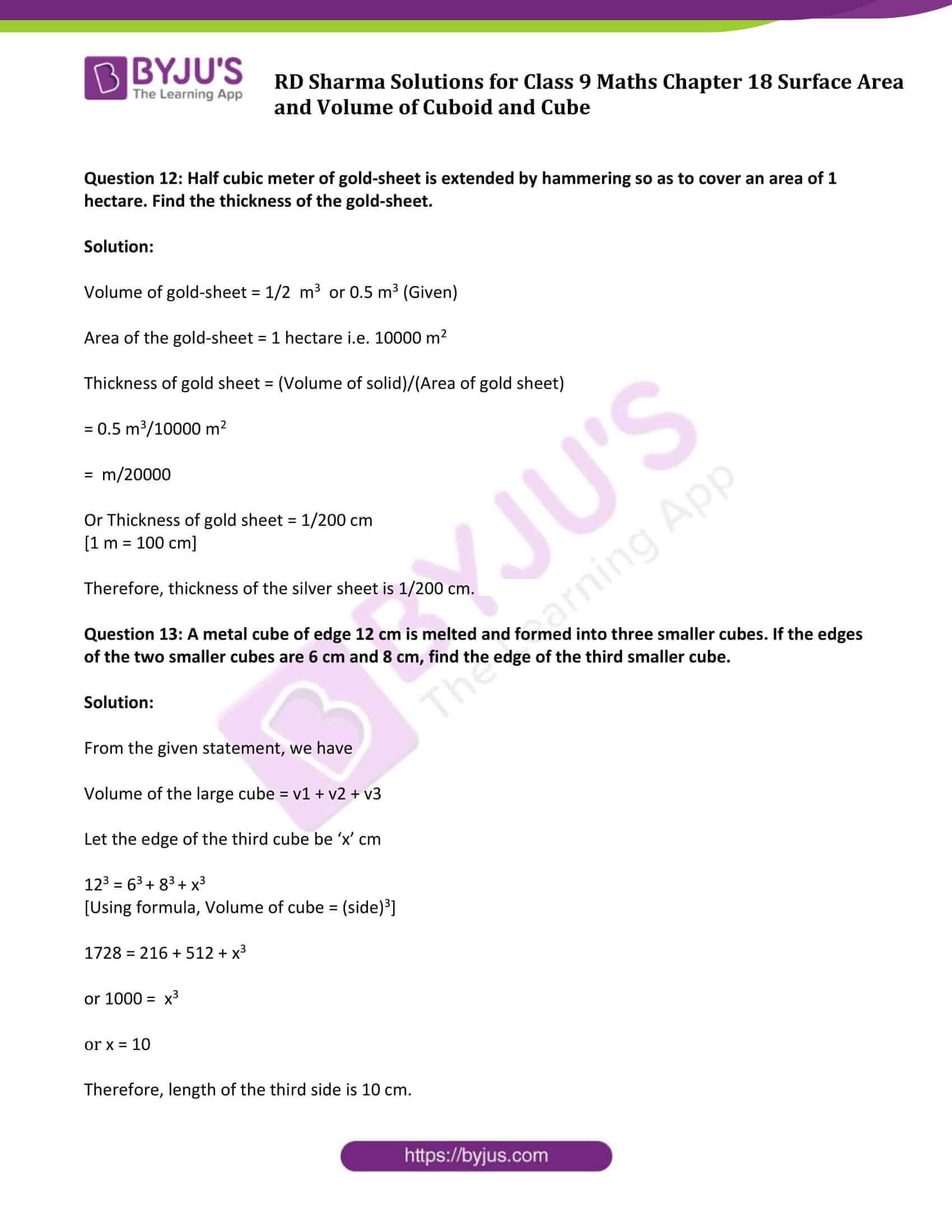### Access Answers to Maths RD Sharma Solutions for Class 9 Chapter 18 Surface Area and Volume of Cuboid and Cube Exercise 18.2 Page number 18.29

Question 1: A cuboidal water tank is 6 m long, 5 m wide and 4.5 m deep. How many liters of water can it hold?

Solution:

Dimensions of a cuboidal water tank:

Length = l = 6m

Height = h = 4.5m

We know, Volume of the cuboidal water tank = lbh

By substituting the values, we get

Volume = 6×5×4.5

= 135

Therefore, Volume of the cuboidal water tank is 135 m3

Convert into liters:

We know; 1 m3 = 1000 liters

So, 135m3 = (135×1000)liters

= 135000 liters

Hence, the tank can hold 1,35,000 liters of water.

Question 2: A cuboidal vessel is 10 m long and 8 m wide. How high must it be made to hold 380 cubic meters of a liquid?

Solution:

Dimensions of a cuboidal vessel:

Length = l = 10 m

Breadth = b = 8 m

Volume of the vessel = 380 m3 (given)

Let ‘h’ be the height of the cuboidal vessel.

We know, Volume of cuboidal vessel = lbh

lbh = 380 m3

or 10×8×h = 380

or h = (380)/(10×8)

or h = 4.75

Therefore, height of the vessel should be 4.75 m.

Question 3: Find the cost of digging a cuboidal pit 8 m long, 6 m broad and 3 m deep at the rate of Rs 30 per m3.

Solution:

Dimensions of a cuboidal pit:

Length = l = 8 m

Breadth = b = 6 m

Depth or height = h = 3 m

We know, Volume of the Cuboidal pit = lbh

= 8×6×3

= 144

Volume of the Cuboidal pit is 144 m3

Now, find the cost:

Cost of digging 1 m3 = Rs. 30 (Given)

Cost of digging 144 m3 = 144 x 30 = Rs. 4320

Question 4: If V is the volume of a cuboid of dimensions a, b, c and S is its surface area, then prove that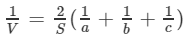Solution:

Dimensions of a cube are:

Length = l = a

Height = h = c

We know, Volume of the cube (V) = lbh

= a×b×c

Or V = abc ….(1)

Again,

Surface area of the cube (S) = 2 (lb+bh+hl)

or S = 2 (ab+bc+ca) ……(2)

Now,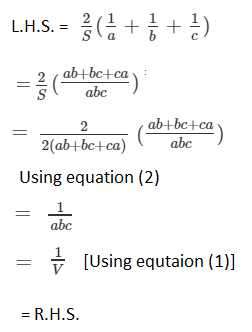Hence Proved.

Question 5: The areas of three adjacent faces of a cuboid are x, y and z. If the volume is V, Prove that V2 = xyz.

Solution:

Let a, b and c be the length, breadth, and height of the cuboid.

Then, x = ab, y = bc and z = ca

[Since areas of three adjacent faces of a cuboid are x, y and z (Given)]

And xyz = ab × bc × ca = (abc)2 ……(1)

We know, Volume of a cuboid ( V ) = abc …..(2)

From equation (1) and (2), we have

V2 = xyz

Hence proved.

Question 6: If the areas of three adjacent face of a cuboid are 8 cm2 , 18 cm2 and 25 cm2. Find the volume of the cuboid.

Solution:

Let x, y, z denote the areas of three adjacent faces of a cuboid, then,

x = l×b = 8 cm2

y = b×h = 18 cm2

z = l×h = 25 cm2

Where l = length of a cuboid, b = breadth of a cuboid and h = height of a cuboid

xyz = 8 × 18 × 25 = 3600 ….(1)

Volume of cuboid (V) = lbh

From above results, we can write,

xyz = lb×bh×lh = (lbh)2 = V2 . …..(2)

Form equation (1) and (2), We get

V2 = 3600

or V = 60

Thus, Volume of the cuboid is 60 cm3

Question 7: The breadth of a room is twice its height, one half of its length and the volume of the room is 512 cu.dm. Find its dimensions.

Solution:

Let, l, b and h are the length, breadth and height of the room.

As per given statement,

b = 2h and b = l/2

⇒ l/2 = 2h

or l = 4h

Now, we have l = 4h and b = 2h

We know, Volume of the room = lbh

Volume of the room = 512dm3 (given)

So, 4h×2h×h=512

or h3=64

or h=4

Therefore, Length of the room (l) = 4h = 4×4 = 16 dm

Breadth of the room (b) = 2h = 2×4 = 8 dm

And Height of the room (h) = 4 dm.

Question 8: A river 3 m deep and 40 m wide is flowing at the rate of 2 km per hour. How much water will fall into the sea in a minute?

Solution:

Water flow of a river = 2 km per hour = (2000/60) m/min or (100/3 )m/min

[we know: 1 km = 1000 m and 1 hour = 60 mins]

Depth of the river (h) = 3m

Width of the river (b) = 40m

Volume of the water flowing in 1 min = 100/3 × 40 × 3 = 4000 m3

Or 4000 m3 = 4000000 litres

Therefore, in 1 minute 4000000 litres of water will fall in the sea.

Question 9: Water in a canal 30 dm wide and 12 dm deep, is flowing with a velocity of 100 km every hour. What much area will it irrigate in 30 minutes if 8 cm of standing water is desired?

Solution:

Water in the canal forms a cuboid of Width (b) and Height (h).

b = 30dm = 3m and h = 12dm = 1.2m

Here, Cuboid length = distance travelled in 30 min with a speed of 100 km per hour.

Therefore, Length of the cuboid (l) = 100 × 30/60 = 50000 metres

Volume of water used for irrigation = lbh = 50000×3×1.2 m3

Water accumulated in the field forms a cuboid of base area equal to the area of the field and height = 8/100 metres (Given)

Therefore, Area of field × 8/100 = 50000 × 3 × 1.2

Area of field = (50000 × 3 × 1.2) × 100/8

= 2250000

Thus, area of field is 2250000 m2 .

Question 10: Three metal cubes with edges 6cm, 8cm, 10cm respectively are melted together and formed into a single cube. Find the volume, surface area and diagonal of the new cube.

Solution:

Let us consider, ‘x’ be the length of each edge of the new cube.

Volume of cube = x3

⇒ x3 = (63 + 83 + 103)cm3

or x3 = 1728

or x=12

Volume of the new cube = x3 = 1728 cm3

Surface area of the new cube = 6(side)2 = 6(12)2 = 864 cm2

And, diagonal of the newly formed cube = √3a = 12√3 cm

Question 11: Two cubes, each of volume 512 cm3 are joined end to end. Find the surface area of the resulting cuboid.

Solution:

Let ‘a’ be the side of a cube.

Volume of the cube = 512cm3 (Given)

We know volume cube = (side)3

⇒ a^3 = 512

or a = 8

Each side of a cube is 8 cm.

Now,

Dimensions of the new cuboid formed are:

Length (l) = 8+8 = 16 cm,

Breadth (b) = 8 cm and

Height (h) = 8 cm

Surface area = 2(lb+bh+hl)

= 2 (16×8+8×8+16×8)

= 640 cm2

Therefore, Surface area of a cube is 640 cm2.

Question 12: Half cubic meter of gold-sheet is extended by hammering so as to cover an area of 1 hectare. Find the thickness of the gold-sheet.

Solution:

Volume of gold-sheet = 1/2 m3 or 0.5 m3 (Given)

Area of the gold-sheet = 1 hectare i.e. 10000 m2

Thickness of gold sheet = (Volume of solid)/(Area of gold sheet)

= 0.5 m3/10000 m2

= m/20000

Or Thickness of gold sheet = 1/200 cm

[1 m = 100 cm]

Therefore, thickness of the silver sheet is 1/200 cm.

Question 13: A metal cube of edge 12 cm is melted and formed into three smaller cubes. If the edges of the two smaller cubes are 6 cm and 8 cm, find the edge of the third smaller cube.

Solution:

From the given statement, we have

Volume of the large cube = v1 + v2 + v3

Let the edge of the third cube be ‘x’ cm

123 = 63 + 83 + x3

[Using formula, Volume of cube = (side)3]

1728 = 216 + 512 + x3

or 1000 = x3

or x = 10

Therefore, length of the third side is 10 cm.

## RD Sharma Solutions for Class 9 Maths Chapter 18 Surface Area and Volume of Cuboid and Cube Exercise 18.2

Class 9 Maths Chapter 18 Surface Area and Volume of Cuboid and Cube Exercise 18.2 is based on the following topics:

• Surface Area of a Cuboid
• Surface Area of a Cube
• Lateral Surface Area of a Cuboid
• Lateral Surface Area of a Cube